# Solar panel series vs parallel: Everything you need to know

In this article, I explain the difference between wiring solar panels in series and wiring them in parallel.

You’ll learn about voltage, current, and power in both configurations, and you’ll also learn how series, parallel, and series-parallel connections are achieved.

## Solar panel series vs parallel: What is the difference?

When you wire solar panels in series or in parallel, you control the voltage (Volts) and current (Amps) of your solar array.

By connecting solar panels in series, you increase the voltage of the system without increasing the current. When you connect solar panels in parallel, you increase the current without increasing the voltage.

In other words, while series-connected solar panels mean higher PV system voltage, parallel-connected solar panels mean higher PV system current.

To understand this better, we’ll use the example of 4 identical solar panels wired in series, parallel, and series-parallel.

### Series connection:

If you connect 4 identical solar panels in series, the total voltage of the string becomes the sum of the voltages of these solar panels, and the current from the string is equal to the current of a single solar panel.Total Voltage (Volts) = Voltage 1 + Voltage 2 + Voltage 3 + Voltage 4

Total Voc (Open-Circuit Voltage) = Voc 1 + Voc 2 + Voc 3 + Voc 4

Total Vmp (Optimum Operating Voltage) = Vmp 1 + Vmp 2 + Vmp 3 + Vmp 4

since all of these voltages are identical, the total voltage is:

Total Voltage (Volts) = 4 x Single Solar Panel Voltage

And the total current is:

Total Current (Amps) = Current 1 = Current 2 = Current 3 = Current 4

Total Isc (Short-Circuit Current) = Isc 1 = Isc 2 = Isc 3 = Isc 4

Total Imp (Optimum Operating Current) = Imp 1 = Imp 2 = Imp 3 = Imp 4

### Parallel connection:

If you connect 4 identical solar panels in parallel, the total voltage is equal to the voltage of a single solar panel, while the total current is the sum of currents from all 4 panels.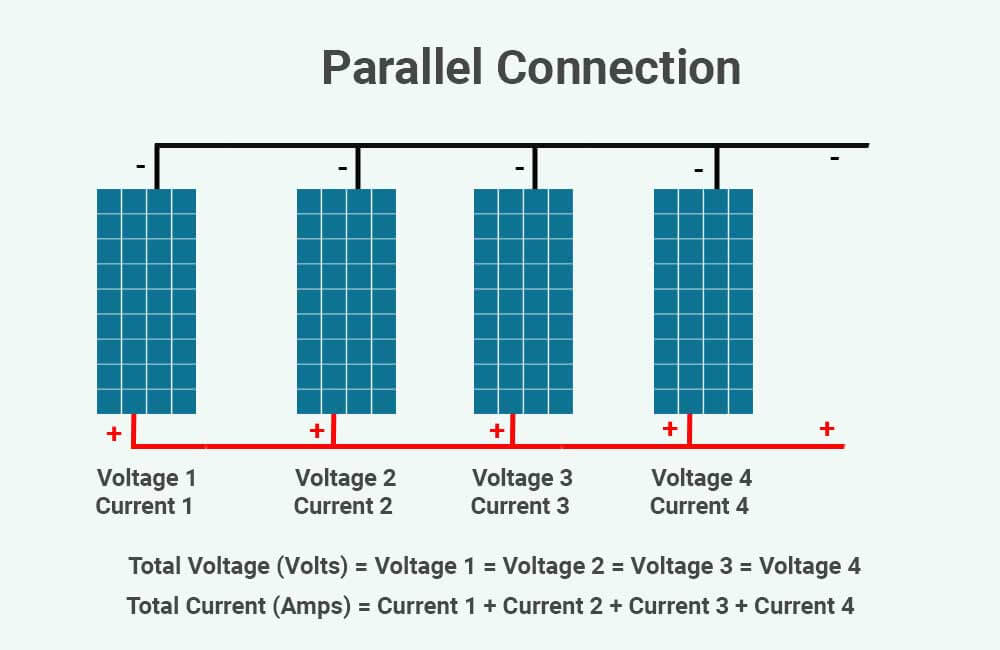Total Voltage (Volts) = Voltage 1 = Voltage 2 = Voltage 3 = Voltage 4

Total Voc (Open-Circuit Voltage) = Voc 1 = Voc 2 = Voc 3 = Voc 4

Total Vmp (Optimum Operating Voltage) = Vmp 1 = Vmp 2 = Vmp 3 = Vmp 4

And the total current is:

Total Current (Amps) = Current 1 + Current 2 + Current 3 + Current 4

Total Isc (Short-Circuit Current) = Isc 1 + Isc 2 + Isc 3 + Isc 4

Total Imp (Optimum Operating Current) = Imp 1 + Imp 2 + Imp 3 + Imp 4

since all of these voltages are identical, the total current is:

Total Voltage (Volts) = 4 x Single Solar Panel Current

### Series/Parallel connection:

This is a type of configuration that has both Parallel and Series connections. Every 2 solar panels are connected in series with each other, forming 2 strings, and the 2 strings are then connected in parallel.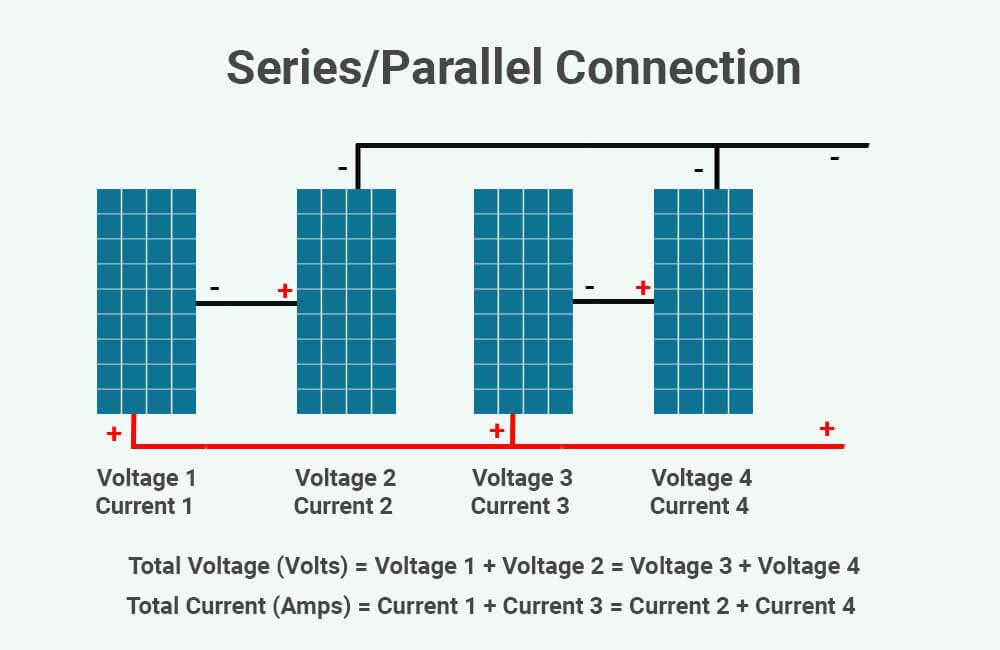In such a setup, the total voltage of the solar array will be equal to the voltage of a single string, and the total current of the solar array will be equal to the sum of the currents of both strings.

Since each string consists of 2 solar panels in series, the voltage of each string is equal to the sum of the voltages of the 2 solar panels:

Total Voltage (Volts) = Voltage of one string = Voltage 1 + Voltage 2 = Voltage 3 + Voltage 4

Total Voc (Open-Circuit Voltage) = Voc 1 + Voc 2 = Voc 3 + Voc 4

Total Vmp (Optimum Operating Voltage) = Vmp 1 + Vmp 2 = Vmp 3 + Vmp 4

The current of each string will be equal to the current of one solar panel, therefore, the total current of the solar array is equal to the sum of the currents of 2 solar panels:

Total Current (Amps) = Current of string 1 + Current of string 2 = Current 1 + Current 3 = Current 2 + Current 4

Total Isc (Short-Circuit Current) = Isc 1 + Isc 3 = Isc 2 + Isc 4

Total Imp (Optimum Operating Current) = Imp 1 + Imp 3 = Imp 2 + Imp 4

### Does connecting solar panels in series increase wattage? or does solar wattage increase in parallel?

When you connect solar panels in series or in parallel the total wattage of the system increases. However, neither of these connections results in a higher system wattage than the other.

For example, if you have 4 identical 100W solar panels and you connect them together. it does not matter if you connect them in series, parallel, or series AND parallel. The result is going to be the same, a 400W solar array.

Wattage or Electrical Power (in Watts), is the product of current and voltage:

Wattage (Watts) = Voltage (Volts) x Current (Amps)

If you have 4 identical solar panels connected in series, the current stays the same as that of a single panel, while the voltage is quadrupled. So the wattage of the solar array becomes:

Wattage (Watts) = Voltage of the solar array (Volts) x Current of the solar array (Amps)

Wattage (Watts) = (4 x Voltage of one solar panel  (Volts)) x Current of one solar panel (Amps)

Wattage (Watts) = 4 x Voltage of one solar panel  (Volts) x Current of one solar panel (Amps)

If you have 4 identical solar panels connected in parallel, the voltage stays the same as that of a single panel, while the current is quadrupled. So the wattage of the solar array becomes:

Wattage (Watts) = Voltage of the solar array (Volts) x Current of the solar array (Amps)

Wattage (Watts) = Voltage of one solar panel  (Volts) x (4 x Current of one solar panel (Amps))

Wattage (Watts) = 4 x Voltage of one solar panel  (Volts) x Current of one solar panel (Amps)

If you have 4 identical solar panels in a series/parallel configuration, the current doubles and the voltage also doubles. The wattage of the solar array becomes:

Wattage (Watts) = Voltage of the solar array (Volts) x Current of the solar array (Amps)

Wattage (Watts) = (2 x Voltage of one solar panel  (Volts) x (2 x Current of one solar panel (Amps))

Wattage (Watts) = 2 x 2 x Voltage of one solar panel  (Volts) x Current of one solar panel (Amps)

Wattage (Watts) = 4 x Voltage of one solar panel  (Volts) x Current of one solar panel (Amps)

So, no matter how you connect these solar panels, in terms of wattage, the end result is going to be the same.

Let’s see how each of these connections is achieved.

## Connecting solar panels in series vs parallel: how is it done?

Solar panels have a special type of connector at their terminals, which is referred to as an MC4 connector:Each solar panel will have a female and a male MC4 connector. Generally, the female MC4 connector represents the negative terminal of the solar panel, and the male MC4 connector represents the positive terminal of the solar panel. However, this isn’t always the case.

In any case, before you make any connections, you’ll need to identify which MC4 connector is the positive and which is the negative.

There are also MC4 branch connectors, which you’ll need if you’re going to have parallel connections in your setup: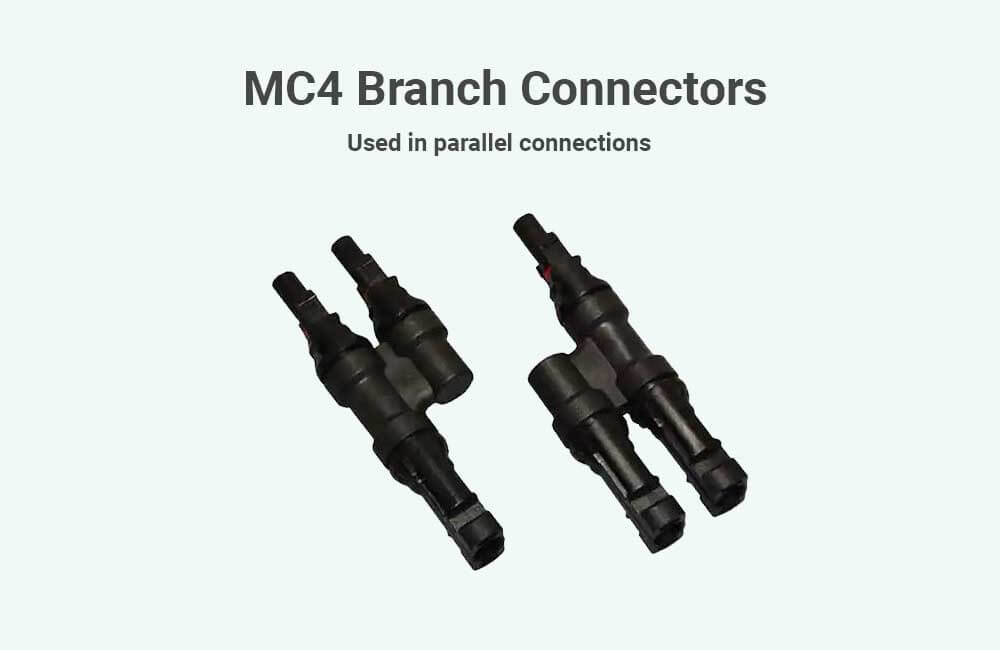The ones in the image above are 2 to 1, but if you have more than 2 solar panels (or more than 2 strings) that’ll be connected in parallel, there are also 3 to 1 or 4 to 1 MC4 branch connectors.

Now let’s see how each type of connection is achieved.

### How to wire solar panels in series?

To wire solar panels in series, connect the positive MC4 connector (male connector for example) of the first solar panel, to the negative MC4 connector of the next solar panel, and so on. When you’re done, you should have 2 unconnected terminals at each end of your string, a positive and a negative.

Here’s an example of 4 solar panels wired in series:The unconnected positive and negative terminals of the string can then be connected to extension cables which will connect the string to the solar charge controller.

Related: What size solar charge controller do I need?

These extension cables will each have an MC4 connector on one end to connect to the solar panels, and stripped on the other end to connect to the solar charge controller.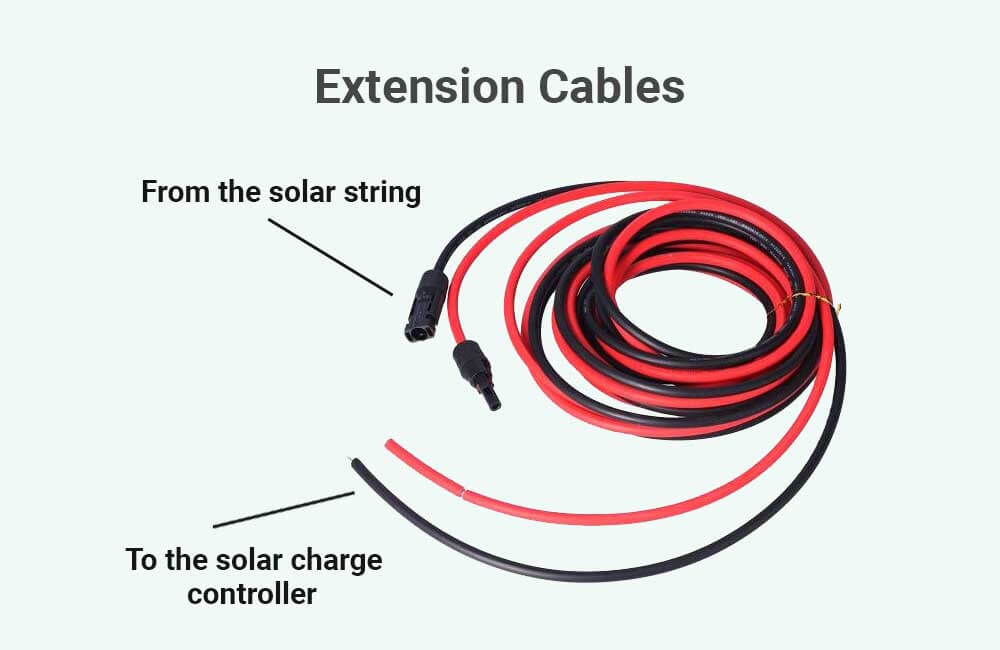### How to wire solar panels in parallel

To wire solar panels in parallel, you’ll need to connect all the positive terminals of the solar panels together to form the positive terminal of the solar array, and all the negative terminals of the solar panels together to form the negative terminal of the solar array. This can be done using MC4 branch connectors.

Here’s an example of 4 solar panels connected in parallel using 4-1 MC4 branch connectors: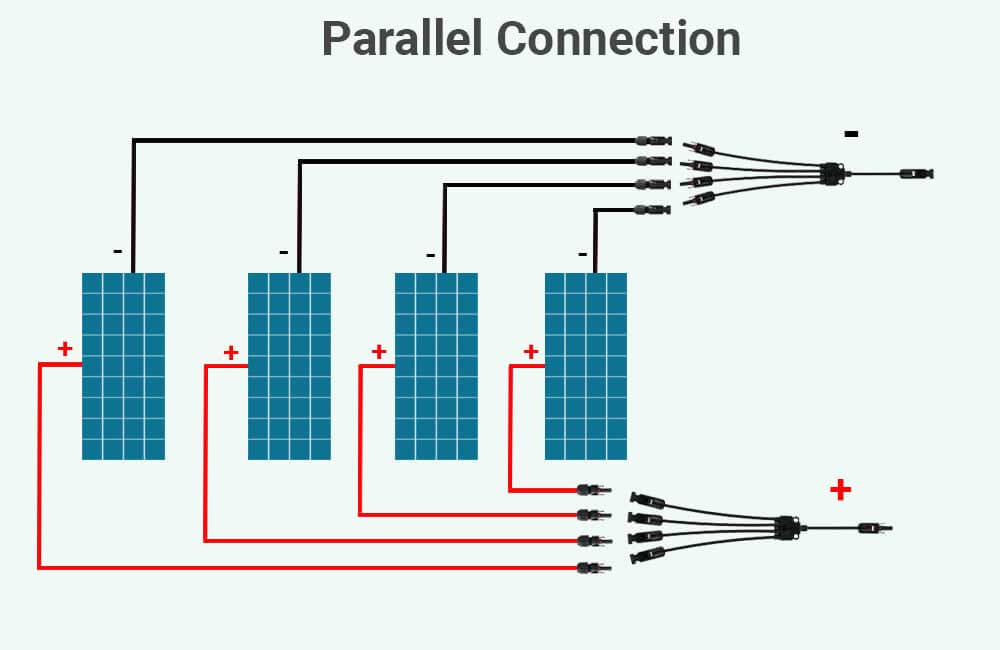The output of each branch connector can then be connected to the extension cables that lead to the solar charge controller.

### How to wire solar panels in series and parallel (series-parallel)

To wire solar panels in series and parallel, wire each string of solar panels in series (positive to negative). Then, using MC4 branch connectors, wire all the positive terminals of the solar strings together, and the negative terminals of the strings together.

For example, how to wire 4 solar panels in series-parallel?

To wire 4 solar panels in a series-parallel configuration, wire every 2 solar panels in series by connecting the positive of one solar panel to the negative of the other solar panel, forming 2 strings. You’ll then need to wire these 2 strings in parallel by connecting their positive terminals together and their negative terminals together.

Here’s an image that illustrates this: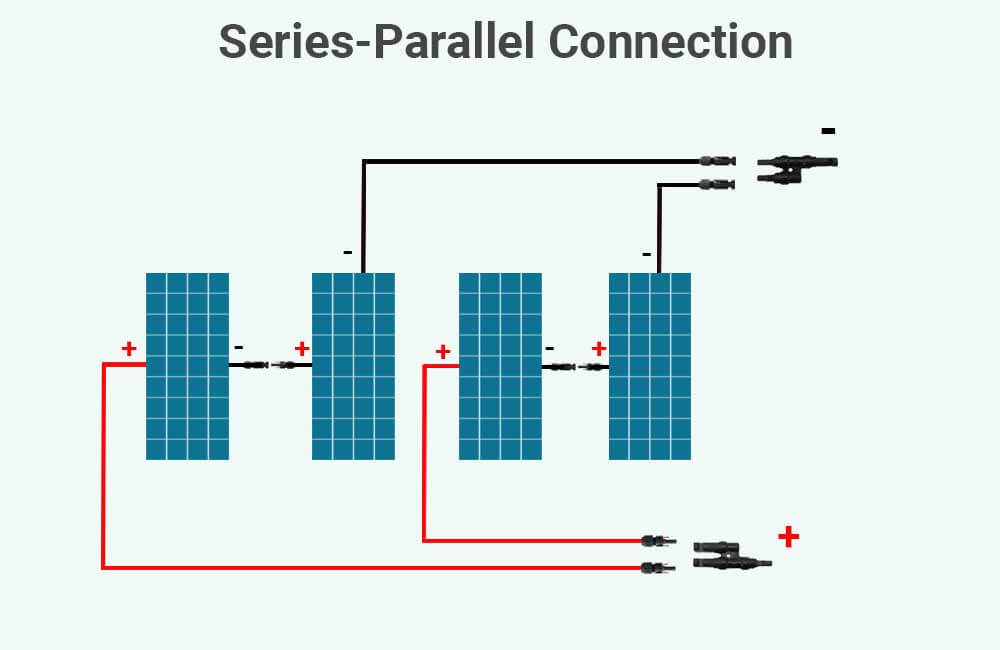## Related Topics:

What size fuse between solar panels and solar charge controller?##### Younes

Hi! My name is Younes. I'm an electrical engineer and a renewable energy enthusiast. I created renewablewise.com with a mission of delivering digestible content and information to the people who seek it.

1. #### Roger Kahrau

You talk about panel output, volts & amps. My question is about the voc voltage, how does that change in series & parallel circuits?

• #### Younes

Hey there Roger,

2. #### richard

whats the best way to wire solar so you can get the maximum out put . i read that in series if the cloud comes over and blocks the first panel you have stuff all power it seems more like every cell has a tap and water flows through all the panels and if a tap is turned off then no water flows to the rest restricting out put . in parallel the out put is not to restricted ? .so is a series parallel system the best way to wire panels or does voltage output come into it and what is the minimum voltage the control unit need

• #### Younes

Hey there Richard,
Please read my article on the effects of shading on solar panels (series vs parallel), I’m sure you’ll find all the info you need there.
Hope this helps.

3. #### Alan Stone

Hi
I have a canal boat with six x 350wat solar panels, and four 12v 300amp lithium batteries. These are connected in a 24v circuit. My BMS shows a 28.40v for all four batteries, but the capacity has gone down from 100% to 35% in the last few weeks with very little use. How can this be with fully charged batteries ?.
Kind regards Alan

• #### Younes

Hey there Alan,
If, in the last few weeks, your solar panels have been getting suboptimal sunlight, and your batteries are sized just enough to offset your daily energy consumption, this seems normal.
So, how much energy (in Watt-hours or kiloWatt-hours) do you usually use on a daily basis?
Also, how many Peak Sun Hours do you get?

4. #### Rank Merida

What happens if 2 panels are connected in series and then connected to one panel in parallel? All panels have the same specs.

• #### Younes

Hello there,
In such a case, the single solar panel will likely be act as a short-circuit due to its bypass diodes. If an MPPT is used, the bypass diodes will not work, and the single panel will end up lowering the combined voltage of the other two panels, which means you’ll have the same power output as if you only had 2 panels in parallel.